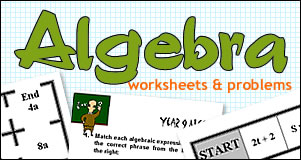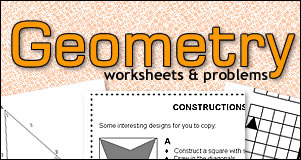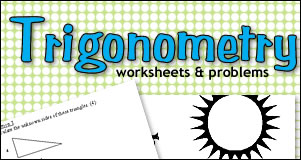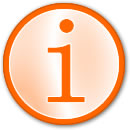# Printable Worksheets & Math Problems

Page updated : 15 April 2018

These math worksheets and math problems are for high school and secondary school math teachers and students.

This is a free database of high school worksheets that are printable directly from your browser for classroom or homework use or for creating lesson plans. Perfect for last minute classroom projects or for relief teachers.

These math problems have been written by teachers from all over the world! And they've been tested in classrooms.

Math worksheets are for classroom use, homework and review. They are for practising math concepts taught in the classroom. For the learning of new math cocepts we suggest you start with our math tutorials.

Click on a topic heading to view a list of available worksheets, math questions and problems.

## HIGH SCHOOL MATH WORKSHEETS## Numeracy Worksheets & Problems

Math problems involving : Numeracy (Addition, Substraction, Multiplication, Division), Fractions, Decimals, Percentages, Ratio, Calculator Skills, Estimation etc## Algebra Worksheets & Problems

Math problems involving : Basic Algebra, Equations, Expressions, Calculus, Graphs etc## Measurement Geometry Worksheets

Math problems involving : Mensuration, Scales and Time, Perimeter, Area, Volume, Emperial & Metric Measurements etc## Geometry Worksheets & Problems

Math problems involving : Euclidian Geometry, Transformation Geometry, Constructions, Loci, Isometrics, Maps and Location, Solids etc## Trigonometry Worksheets & Problems

Math problems involving : Pythagorus, Trigometric Ratios, Sine and Cosine Rules, 3D Trigonometry etc## Statistics Worksheets & Problems

Math problems involving : Statistics, Investigations, Recording, Analysing and Displaying Data, Probability etc

##A note about year levels

Where appropriate each worksheet is given a year level that it is applicable to. As we're all in different countries the year level corresponds to the number of years at school. So, for example, a worksheet for Year 11 is for students in their 11th year of school.
Worksheets for earlier or later years may still be suitable for you.

## HIGH SCHOOL MATH RESOURCES## Math Review Tutorials

Need help with your math?

Try our math tutorials. Lots of online high school math problems for a range of subjects.

Each math review has worked examples and math problems for you to try. This is a free resource for high school math students and for parents who would like to help your child learn math.

## FUN MATH

Learn math concepts and practise your math skills with games and puzzles.## Cool Math Games

You can have fun while learning high school math. Visit our cool math games section for a great way to review your math. Review, learn, develop logic and spatial skills. Exercise the math side of your brain.## High School Math Puzzles

Math puzzles can be very unusual and entertaining. Math can be more interesting than expected! Various online puzzle games here, as well as high school math games & puzzles worksheets.## Fun Math Projects

Teachers! Looking for high school math activities and projects for inspire your math students? Try our fun math projects.

• Math for Valentine's Day
• Math and Art
• Math in Nature
• and more...Please note : This is a free service and these worksheets and problems are supplied on 'as is' basis. We will not enter into any correspondence on the content of the worksheets and problems, errors, answers or tuition.